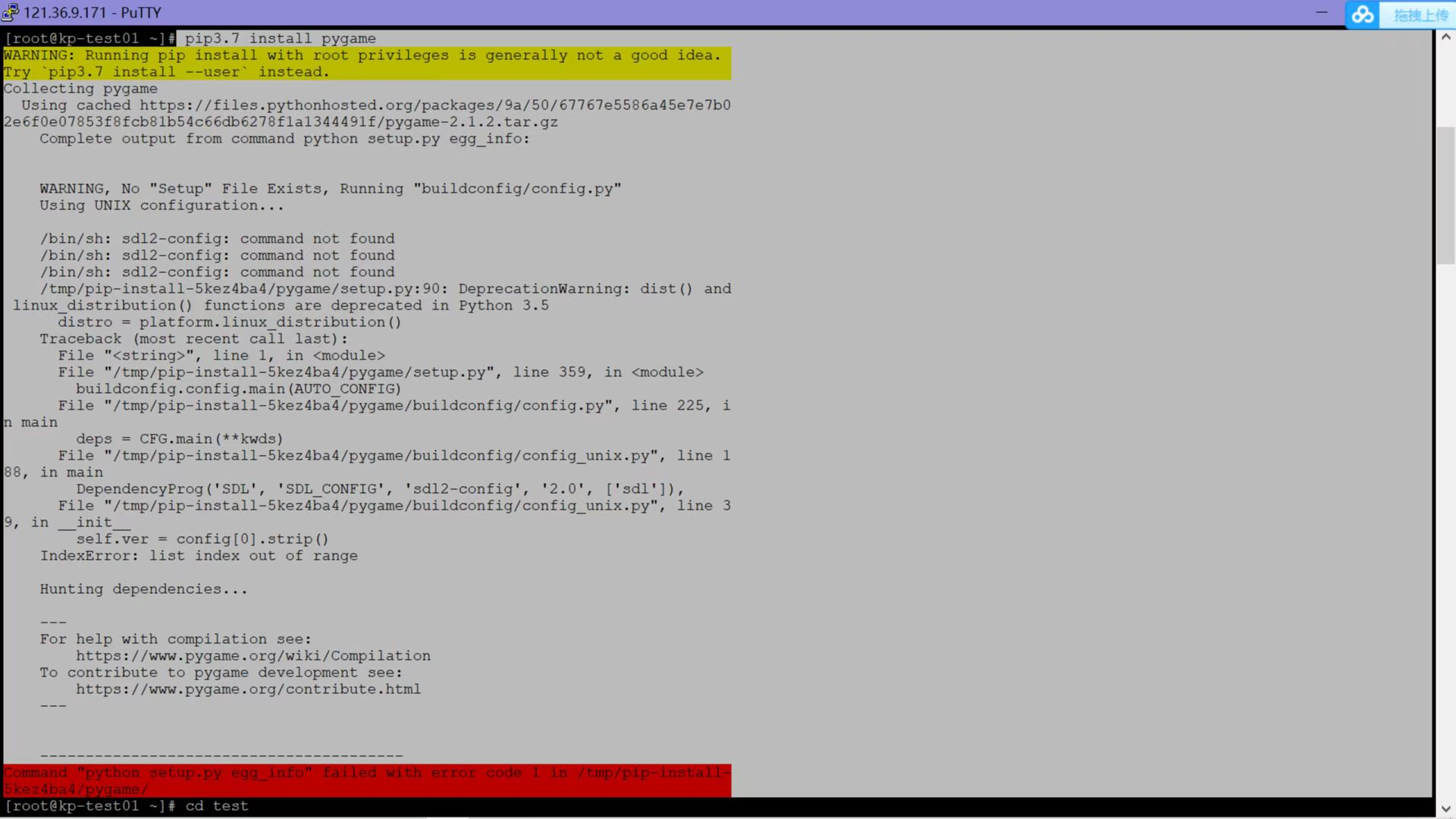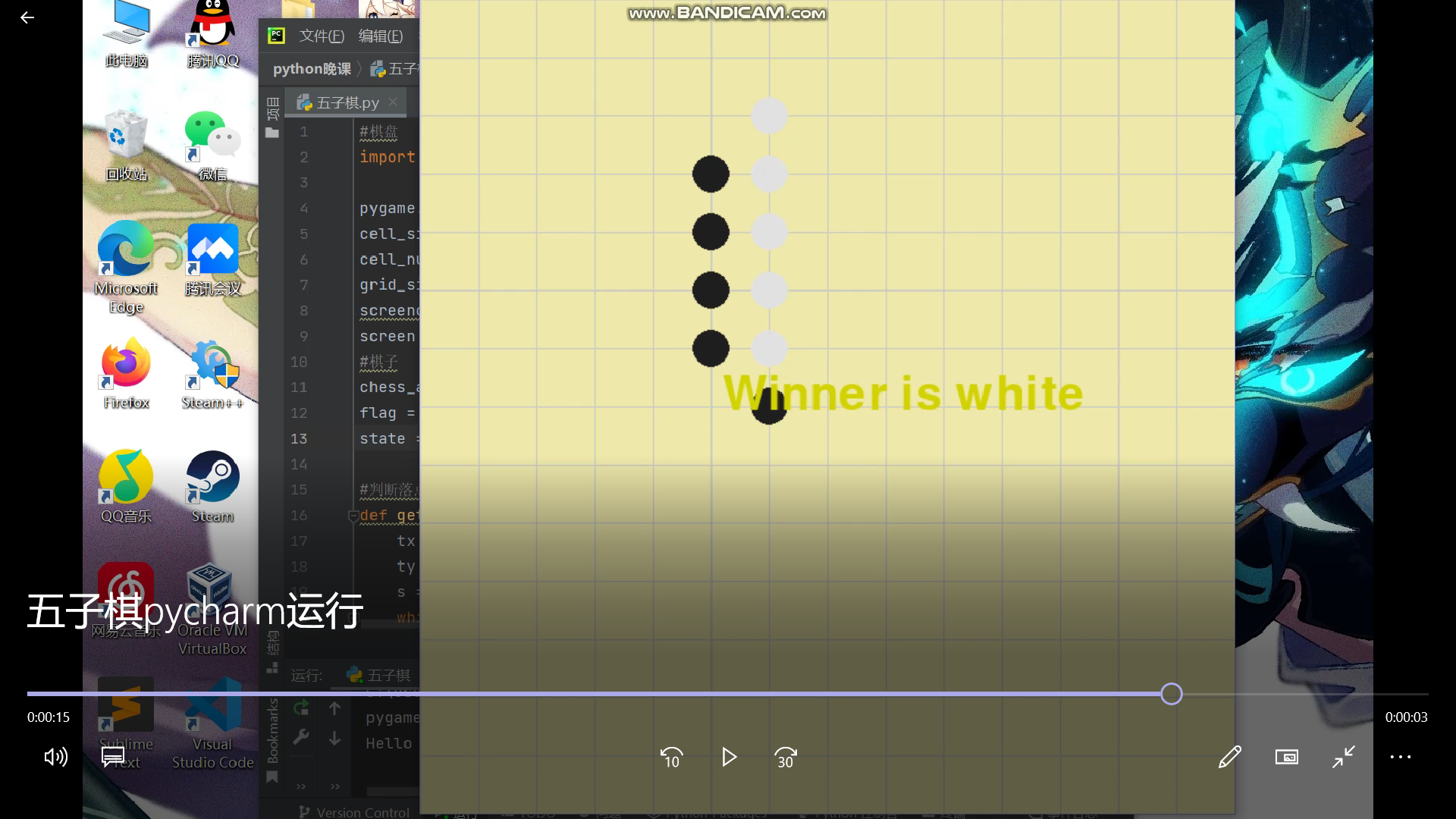# 20212323 2021-2022-2《Python程序设计》实验四报告

## 1. 实验内容

Python综合应用：爬虫、数据处理、可视化、机器学习、神经网络、游戏、网络安全等。课代表和各小组负责人收集作业（源代码、视频、综合实践报告）

## 2. 实验过程及结果

### （2） 实验设计

* import pygame
pygame.init()
cell_size = 50
cell_num = 15
grid_size = cell_size * (cell_num - 1)
screencaption = pygame.display.set_caption('五子棋')
screen = pygame.display.set_mode((grid_size, grid_size))

chess_arr = []
flag = 1
state = 1

def get_one_dire_num(lx, ly, dx, dy, m):
tx = lx
ty = ly
s = 0
while True:
tx += dx
ty += dy
if tx < 0 or tx >= cell_num or ty < 0 or ty >= cell_num or m[ty][tx] == 0: return s
s += 1

def check_win(chess_arr, flag):
m = [ * cell_num for i in range(cell_num)]
for x, y, c in chess_arr:
if c == flag:
m[y][x] = 1
lx = chess_arr[-1]
ly = chess_arr[-1]
dire_arr = [[(-1, 0), (1, 0)], [(0, -1), (0, 1)], [(-1, -1), (1, 1)],
[(-1, 1), (1, -1)]]

for dire1, dire2 in dire_arr:
dx, dy = dire1
num1 = get_one_dire_num(lx, ly, dx, dy, m)
dx, dy = dire2
num2 = get_one_dire_num(lx, ly, dx, dy, m)
if num1 + num2 + 1 >= 5:
return True

return False

while True:
for event in pygame.event.get():
if event.type == pygame.QUIT:
pygame.quit()
exit()

if state == 1 and event.type == pygame.MOUSEBUTTONUP:
x, y = pygame.mouse.get_pos()
xi = int(round((x) * 1.0 / cell_size))
yi = int(round((y) * 1.0 / cell_size))
if xi >= 0 and xi < cell_num and yi >= 0 and yi <
cell_num and (xi, yi, 1) not in chess_arr and (
xi, yi, 2) not in chess_arr:
chess_arr.append((xi, yi, flag))
if check_win(chess_arr, flag):
state = 2 if flag == 1 else 3
else:
flag = 2 if flag == 1 else 1
screen.fill((238, 232, 170))
for x in range(0, cell_size * cell_num, cell_size):
pygame.draw.line(screen, (200, 200, 200), (x, 0 ),
(x, cell_size * (cell_num - 1)), 1)
for y in range(0, cell_size * cell_num, cell_size):
pygame.draw.line(screen, (200, 200, 200), (0, y),
(cell_size * (cell_num - 1), y), 1)

for x, y, c in chess_arr:
chess_color = (30, 30, 30) \
if c == 1\
else (225, 225, 225)
pygame.draw.circle(screen, chess_color, [x * cell_size, y * cell_size], 16, 16)

if state != 1:
myfont = pygame.font.Font(None, 60)
white = 210, 210, 0
win_text = "Winner is %s" % ('black' if state == 2 else 'white')
textImage = myfont.render(win_text, True, white)
screen.blit(textImage, (260, 320))

pygame.display.update()


### （3） 实现过程### （4） 实验结果## 3. 实验过程中遇到的问题和解决过程

• 问题1：设计时考虑不全面，对棋盘大小，棋子大小等没有清晰确定的方案
• 解决：一次一次的尝试，不断修改
• 问题2：不会用pygame
• 解决：结合《Python编程：从入门到实践》一书，参考CSDN、博客园里的大佬们的教学，自己慢慢摸索
• 问题3：在华为云服务器（ECS）中用putty、WinSCP运行时，出现很多问题，比如putty终端不能显示游戏画面、没有pygame运行不了
• 解决：在其他同学的帮助下，下载了Xming等一系列工具，使后续的游戏画面能够得以显示，但是始终下载不了pygame导致直接运行不了，问题未能解决。

## 4. 学习总结及心得

• 学习总结
经过一个学期的python学习，我了解了很多，从最开始初步了解python，到学习python语言基础，再到流程控制语句、序列、字符串、正则表达式、函数、面向对象程序设计、文件操作和异常处理、数据库操作、爬虫等复杂的部分，短短的时间内感觉自己提升了许多，深深的感觉到编程的魅力。或许有的内容运用得还不是很熟练，但我相信python一定会伴随我以后的生活。
• 学习心得
在学习Python之前，我从来没了解、学习过编程语言，，只在上个学期《网络空间安全专业导论》的课程学习中自学过一点Python基础知识，从那时起，我就感觉好像发现了一片新大陆，那种激动、神奇的心情是无以言表的。在《Python程序设计》课程的学习过程中，我又不断接受到更多更深层次的知识，很难想象到开发编程语言的前辈们是多么的聪慧。Python最吸引我的点我觉得应该是它经过一些人的努力被开发出来，接着又有一代又一代的人们去探索、发现不足并不断修补增添，小部分的人努力做出来一个完整的语言系统后，用它来服务大部分的人，对我来说，这个过程极具魅力。在跟着王志强老师不断学习Python的过程中，我也遇到许多抽象、难以理解的东西，甚至陷入迷茫，完全不知道这一部分内容是用来干嘛的，但在跟着老师敲代码的时候，又逐渐理解了，一块知识点是死的，但是当你敲代码时，却能够灵活运用它。一个很复杂很长的代码也能在不断优化之后变为短短几行，这也是需要不断深入学习之后才能够做到的，我现在的“道行”还不深，我会不断学习，努力提升自己。
人生苦短，我学Python！
posted @ 2022-05-31 19:16  20212323  阅读(15)  评论(0编辑  收藏  举报